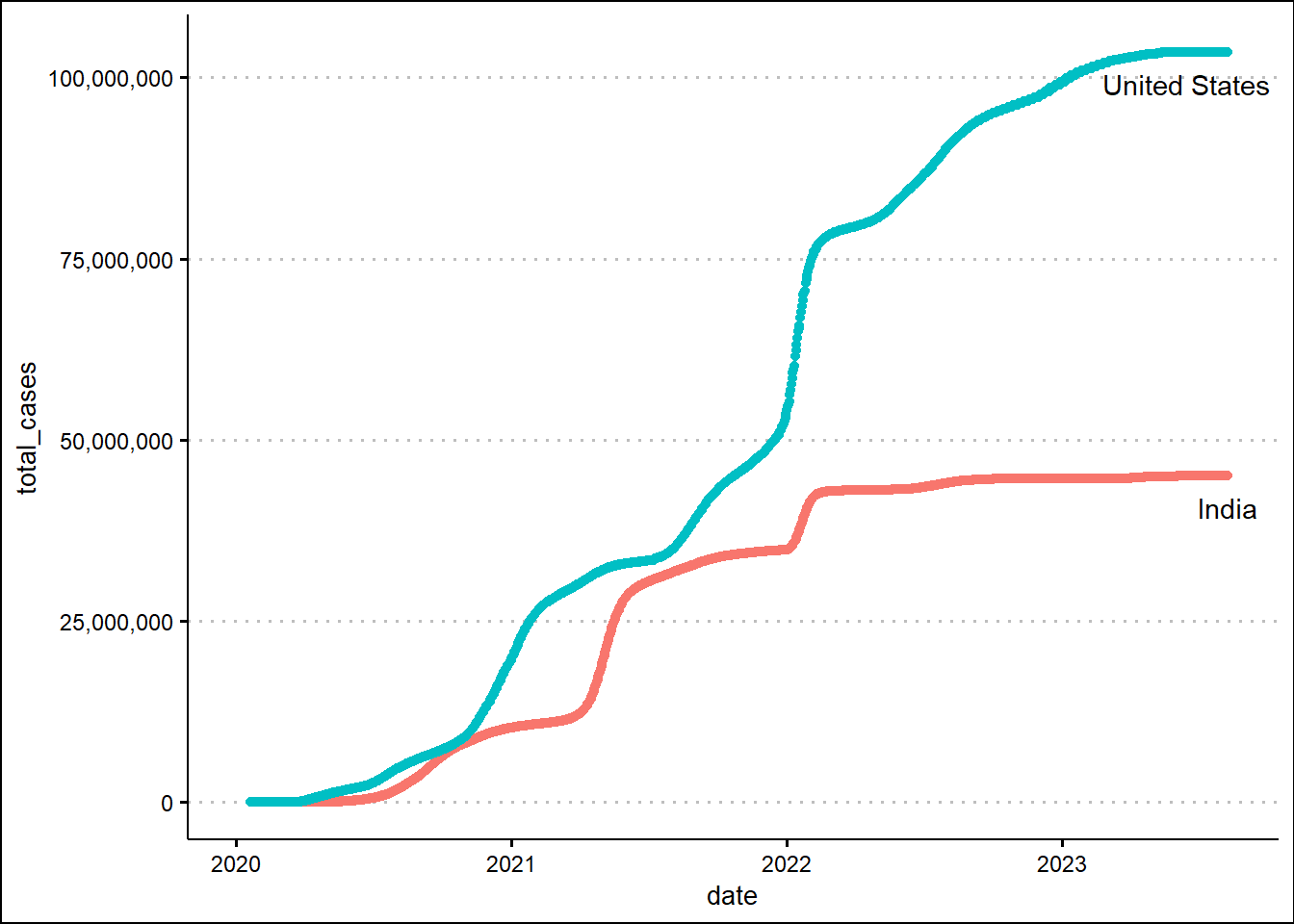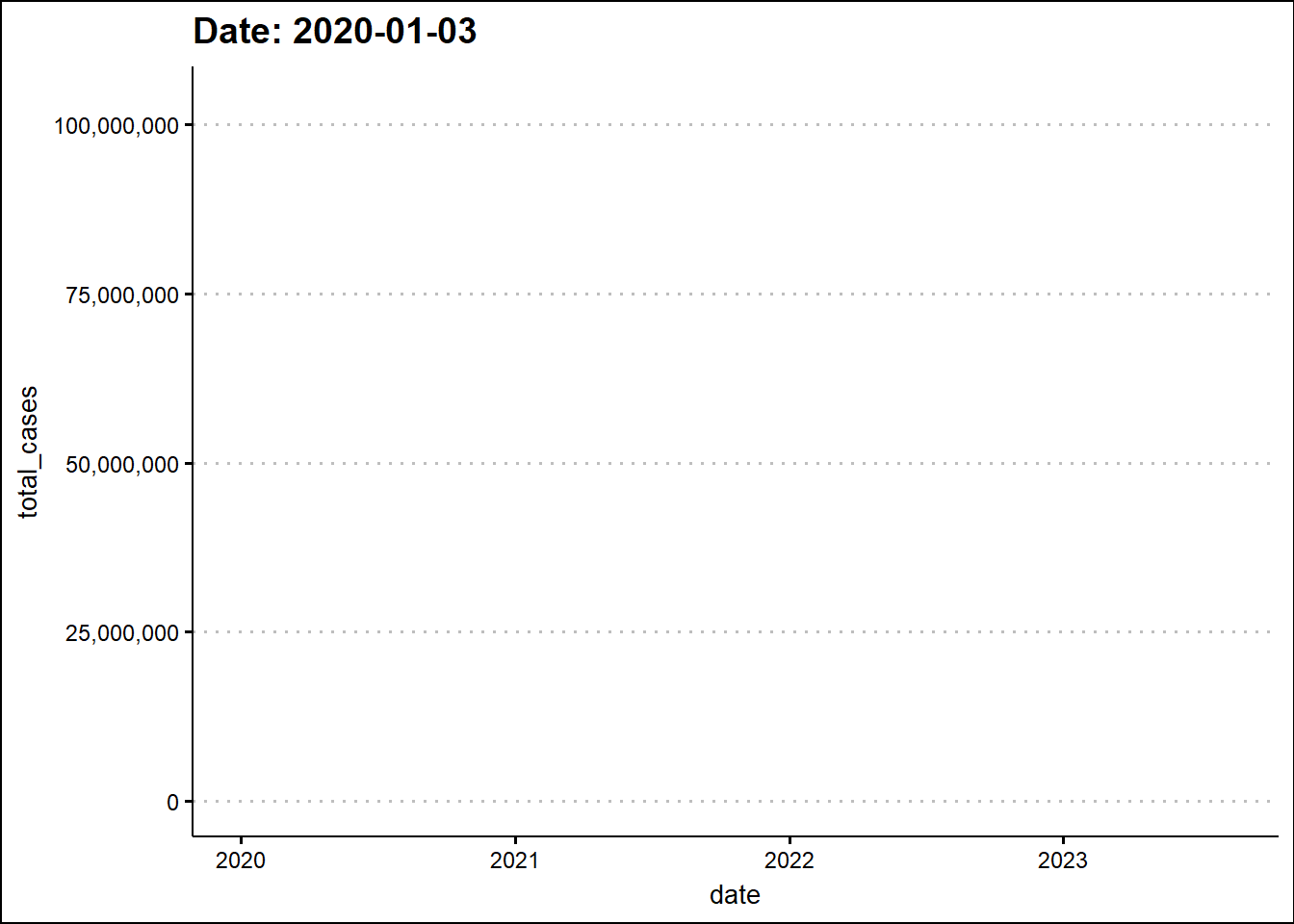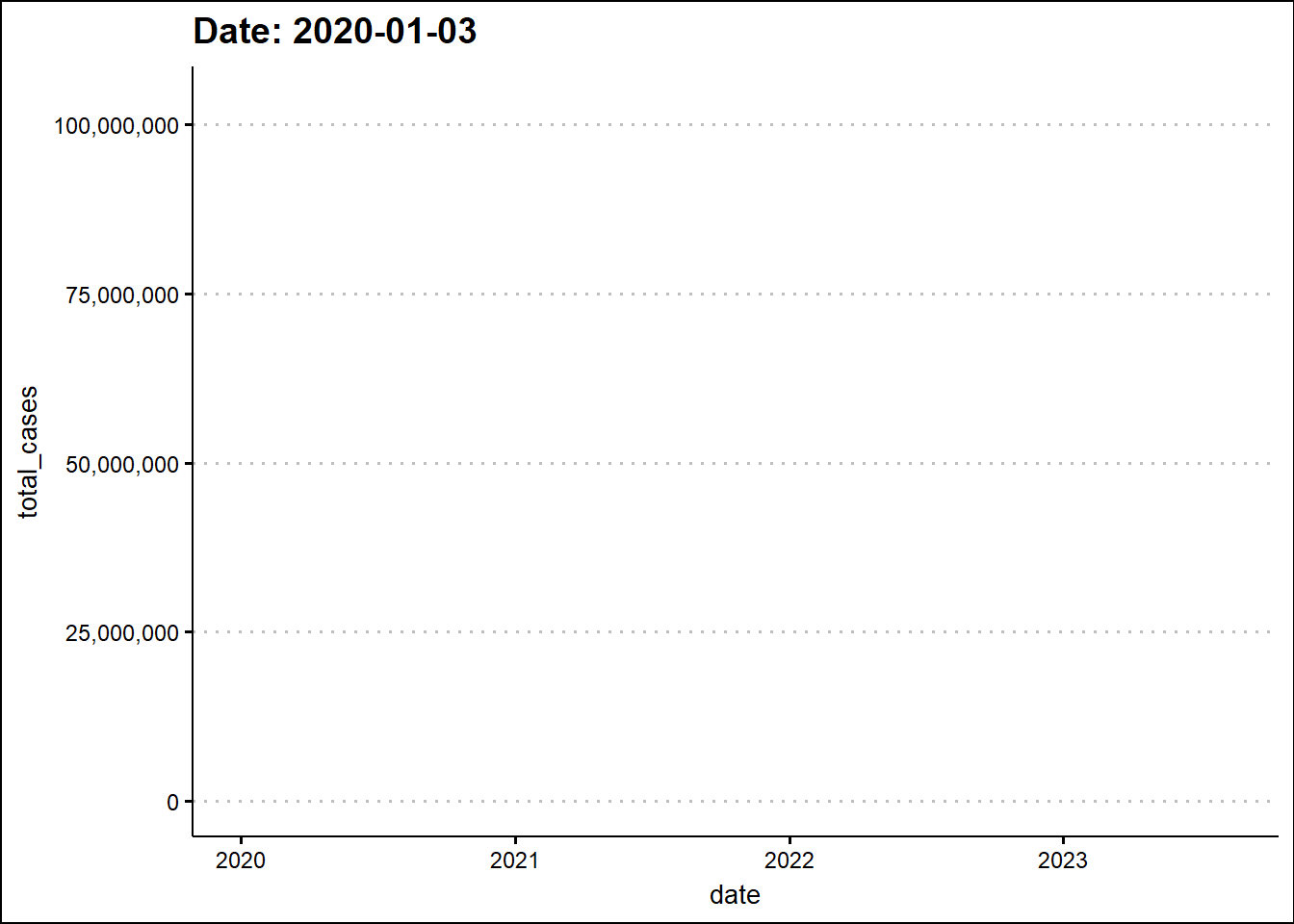# Animated graphs in R

gganimate in action
R
ggplot2
animation
Author

Manish Datt

Published

August 17, 2023

Effective data visualization is at the cornerstone of data science research. The R programming language offers a robust plotting library - `ggplot2`. It is based on the tenets of grammar of graphics which imply that a graph is generated using layers of information including data, coordinates, and representations. We can further enhance the information conveyed by a graph by adding appropriate animations. The `gganimate` library has some useful functions that make it a breeze to animate graphs in R.

To learn how to make animated graphs, we'll use the Covid19 data from our world in data. First, let's make a simple plot showing the timeline of the total number of cases for India and the United States. We’ll make use of the `tibble` and `dplyr` libraries to parse the csv file having the Covid19 data.

``````library(tibble)
library(dplyr)
``df_covid``
``````# A tibble: 332,467 × 67
iso_code continent location    date  total_cases new_cases new_cases_smoothed
<chr>    <chr>     <chr>       <chr>       <dbl>     <dbl>              <dbl>
1 AFG      Asia      Afghanistan 2020…          NA         0                 NA
2 AFG      Asia      Afghanistan 2020…          NA         0                 NA
3 AFG      Asia      Afghanistan 2020…          NA         0                 NA
4 AFG      Asia      Afghanistan 2020…          NA         0                 NA
5 AFG      Asia      Afghanistan 2020…          NA         0                 NA
6 AFG      Asia      Afghanistan 2020…          NA         0                  0
7 AFG      Asia      Afghanistan 2020…          NA         0                  0
8 AFG      Asia      Afghanistan 2020…          NA         0                  0
9 AFG      Asia      Afghanistan 2020…          NA         0                  0
10 AFG      Asia      Afghanistan 2020…          NA         0                  0
# ℹ 332,457 more rows
# ℹ 60 more variables: total_deaths <dbl>, new_deaths <dbl>,
#   new_deaths_smoothed <dbl>, total_cases_per_million <dbl>,
#   new_cases_per_million <dbl>, new_cases_smoothed_per_million <dbl>,
#   total_deaths_per_million <dbl>, new_deaths_per_million <dbl>,
#   new_deaths_smoothed_per_million <dbl>, reproduction_rate <dbl>,
#   icu_patients <dbl>, icu_patients_per_million <dbl>, hosp_patients <dbl>, …``````
``````library(ggplot2)
library(ggthemes)

countries <- c("India", "United States")
## maximum number of cases for countries.
max_vals <- df_covid %>%
group_by(location) %>%
filter(location %in% countries) %>%
slice(which.max(total_cases)) %>%
pull(total_cases)

last_date = tail(df_covid\$date,1)

df_covid %>%
mutate(date = as.Date(date)) %>%
group_by(location) %>%
filter(location %in% countries) %>%
ggplot(aes(x=date, y=total_cases, color=location)) + geom_point() +
scale_y_continuous(labels = scales::comma) +
annotate("text", label=countries, x=as.Date(last_date),y=max_vals, vjust=2) +
annotate("text", label=countries, x=as.Date(last_date),y=max_vals, vjust=2, hjust=0.75) +
theme_clean() + theme(legend.position = "none")``````Next, we'll animate the timeline using the `transition_reveal` function from the `gganimate` library.

``````library(gganimate)

df_covid %>%
filter(location %in% c("India", "United States")) %>%
mutate(date = as.Date(date)) %>%
ggplot(aes(x=date, y=total_cases, color=location, label=location)) + geom_line() +
scale_y_continuous(labels = scales::comma) +
theme_clean() + theme(legend.position = "none") +
labs(title = 'Date: {frame_along}') +
transition_reveal(date) +
geom_text(aes(label=location, group=location))``````
```````geom_line()`: Each group consists of only one observation.
ℹ Do you need to adjust the group aesthetic?``````To enhance the information in the graph, we’ll make a scatter plot and change the marker size based on daily new cases.

Show the code
``````df_covid %>%
filter(location %in% c("India", "United States")) %>%
mutate(date = as.Date(date)) %>%
ggplot(aes(x=date, y=total_cases, size=new_cases, color=location)) +
geom_point() +
scale_y_continuous(labels = scales::comma) +
theme_clean() + theme(legend.position = "none") +
labs(title = 'Date: {closest_state}') +
transition_states(date) +
ease_aes('linear') +